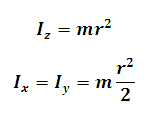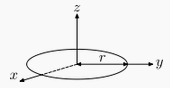# Moment of Inertia of a Ring Calculator

This Calctown Calculator calculates the Moment of Inertia of a Ring about a Perpendicular and Planar axis passing through the center of the ring.

kg
m

#### Result

kg-m2
kg-m2Click here to view image

where

Iz = moment of inertia about perpendicular axis of rotation

Ix = Iy = moment of inertia about planar axis of rotation

m = mass of the ring

r = radius of the ring